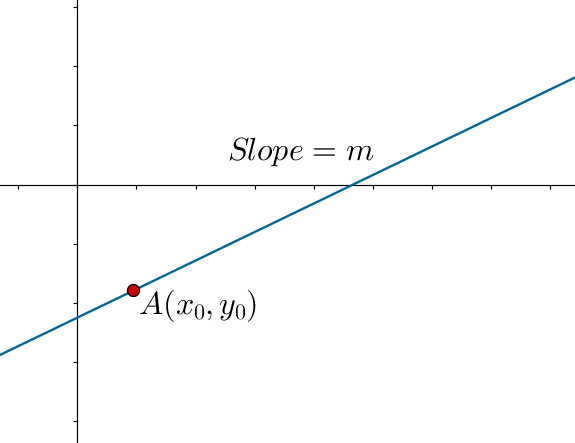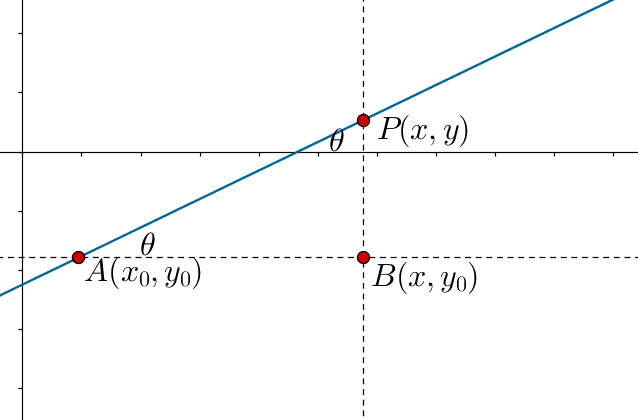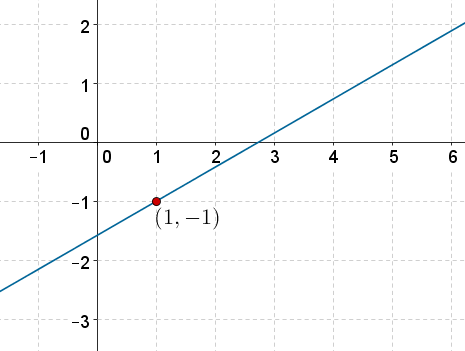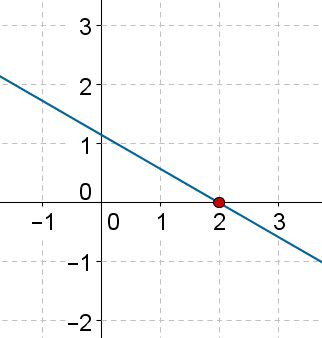# Point-Slope Form

Go back to  'Coordinate-Geometry'

Suppose that we are given the slope m of a line, and one point $$A\left( {{x_0},{y_0}} \right)$$ lying on it:Clearly, the line is uniquely determined. How can we find the equation of the line in such a scenario?

Let $$P\left( {x,y} \right)$$ be any point lying on this line. Observe the following figure carefully:Clearly, $$\angle PAB = \theta$$ , and so

\begin{align}&\tan \theta = m = \frac{{PB}}{{AB}}\\&\Rightarrow \,\,\,m = \frac{{y - {y_0}}}{{x - {x_0}}}\\&\Rightarrow \,\,\,y - {y_0} = m\left( {x - {x_0}} \right)\end{align}

Thus, the equation of a line with slope m and passing through the point $$A\left( {{x_0},{y_0}} \right)$$ is

$y - {y_0} = m\left( {x - {x_0}} \right)$

This is known as the point-slope form of a line’s equation, as we have used the slope of the line and a point lying on the line to specify it.

Example 1: A line is inclined at an angle of 300 to the horizontal, and passes through the point $$\left( {1, - 1} \right)$$ . Determine its equation.

Solution: The following figure depicts this line:We have:

$m = \tan {30^0} = \frac{1}{{\sqrt 3 }}$

Using the point-slope form, the equation of the line is

\begin{align}&y - {y_0} = m\left( {x - {x_0}} \right)\\&\Rightarrow \,\,\,y - \left( { - 1} \right) = \frac{1}{{\sqrt 3 }}\left( {x - 1} \right)\\&\Rightarrow \,\,\,y + 1 = \frac{1}{{\sqrt 3 }}\left( {x - 1} \right)\\&\Rightarrow \,\,\,\sqrt 3 y + \sqrt 3 = x - 1\\&\Rightarrow \,\,\,x - \sqrt 3 y - 1 - \sqrt 3 = 0\end{align}

Example 2: What is the y-intercept of the line which is inclined at an angle of 1500 with the horizontal and passes through the point $$\left( {2,0} \right)$$?

Solution: The following figure depicts this line:We have:

$m = \tan {150^0} = - \tan {30^0} = - \frac{1}{{\sqrt 3 }}$

The equation of the line using the point-slope form is

\begin{align}&y - {y_0} = m\left( {x - {x_0}} \right)\\&\Rightarrow \,\,\,y - 0 = - \frac{1}{{\sqrt 3 }}\left( {x - 2} \right)\\&\Rightarrow \,\,\,y = \left( { - \frac{1}{{\sqrt 3 }}} \right)x + \left( {\frac{2}{{\sqrt 3 }}} \right)\end{align}

Clearly, the y-intercept is

$c = \frac{2}{{\sqrt 3 }}$

Coordinate Geometry
Grade 9 | Questions Set 1
Coordinate Geometry
Coordinate Geometry
Grade 10 | Questions Set 1
Coordinate Geometry